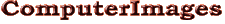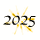Support# Excel’s IF Function.

Switches are among a railroad’s most useful tools — they let a dispatcher send a train over one of several tracks. Similarly Excel users sometimes want a calculation to apply one equation in certain circumstances and a different one in others. A bonus, for example, may be computed one way for long-time employees and differently for others. Calculations like that are sometimes said to have conditional results and the IF function is the tool for making them in Excel.

The Basics. Like a railway switch, Excel’s IF function sends a calculation in one of two directions. The choice depends on the answer to a true/false question, which Microsoft calls a “logical test.” One equation is applied when the answer to the question is TRUE; another when it’s FALSE. It looks like this:

= IF ( logical test , value if TRUE , value if FALSE )

Like all Excel functions, the IF function has certain rules:

• Its name must be spelled correctly (capitalization doesn’t matter);
• The name must be followed by parentheses;
• The information the function uses (called its arguments) goes inside the parentheses; and
• The arguments must be separated by commas.

For example, suppose a company pays a \$1,000 bonus to employees with more than 3 years of service and \$500 to everyone else. Assuming the number of years is entered in cell A2, the following formula calculates the bonus:

= IF(A2>3, 1000, 500)

Formulating the Question. This is critical since Excel applies the logical test exactly as written. Our example with the condition A2>3 gives a person with exactly 3 years service a \$500 bonus. If that person is entitled to \$1000, the condition must say A2>=3. Figure 1 lists Excel’s comparison operators.

Testing Text Entries. To test whether a cell contains certain characters, the character string must be enclosed by quotation marks. For example, suppose our bonus is changed so that \$1000 is paid to people who are coded F because they work overseas. The test A3="F" checks whether the value of cell A3 is the letter F. This test is not case-sensitive, so F and f both produce a true result.

To make the condition case-sensitive, the question itself must contain a function, in this case EXACT. The EXACT function produces a TRUE result when two items are identical. Thus EXACT(A3, "F") is TRUE when A3 contains F and FALSE for f. The final formula looks like this:

= IF(EXACT(A3, "F"), 1000, 500)

Note that the EXACT function has its own parentheses and comma between the arguments.

Multi-part Questions. Sometime the test has more than one condition. For example, suppose our bonus plan is changed so that both years of service and overseas work are considered in determining the amount.

To write the the formula we need to know exactly how the conditions are applied: in our case does a person have to meet BOTH the years of service and overseas conditions to get the larger bonus, or is either one alone enough? If BOTH conditions are required (A2>3 and A3="F") we need the AND function and formula would be:

= IF((AND(A2>3, A3="F"), 1000, 500)

On the other hand, if a person only needs to meet ONE of the conditions (A2>3 or A3="F"), OR is the appropriate function and the formula is:

= IF((OR(A2>3, A3="F"), 1000, 500)

The AND and OR functions can have up to 255 conditions.

NOT. Sometimes its easier to list the few items we don’t want than the many we do. For example, we might want to charge a \$50 fee to all customers outside Massachusetts. It would be difficult to list every other state, plus all foreign countries and territories. Instead, if the state is in A4 we can write:

= IF(NOT(A4="Massachusetts"), 50, 0)

In this example the NOT function doesn’t really simplify things since we could get the same result simply by reversing the true and false results, = IF(A4="Massachusetts", 0, 50). But in more complex equations using the NOT function in combination with AND and OR enables us to express conditions that otherwise would be difficult to write.

Specifying the Results. The TRUE and FALSE results in our bonus example are simple numbers, 1000 and 500. Either one, however, can be a formula and include functions. For example, our bonus for people with more than 3 years of service might be changed to \$500 for every year. The formula for that would be (assuming the number of years is in A2):

= IF(A2>3, 500*A2, 500)

Equations for TRUE and FALSE results can also include functions. A salesperson’s bonus might be based on sales. In that case either the TRUE or FALSE result could include a SUM function that computed the total from the person’s sales history.

Multiple IFs. A common question is how does this work where there are more than two choices? The answer is nested IFs.

Say our bonus example is changed so that people with more than 5 years of service get \$500 per year. Those with more than 3 but not more than 5 get \$1000 and everyone else gets \$500. The following would do the trick:

= IF(A2>5, 500*A2, IF(A2>3, 1000, 500))

When A2>5 Excel applies the TRUE result, 500*A2, and the calculation is done. When A2 is not greater than 5, the FALSE result, IF(A2>3, 1000, 500), is used to award 1000 or 500 based on the number in A2.

Excel 2016 introduced a new function, IFS, which simplifies the process of writing nested IFs. Essentially it is a series of conditions, each of which is followed by a TRUE result. Excel starts from the left and evaluates each condition until it finds one that is TRUE. The formula looks like this for our example:

= IFS(A2>5, 500*A2, A2>3, 1000, TRUE, 500)

Here the last condition is TRUE, so anyone who doesnt qualify for the 3 year or 5 year bonus receives \$500. Without that the result for those people would be #N/A.

SUMIF, COUNTIF. Functions like SUMIF, COUNTIF and AVERAGEIF combine the conditional tests of the IF function with calculations like SUM, COUNT and AVERAGE. Figure 3 shows SUMIF being used to summarize sales by state in Column E based on sales numbers in Column B and state codes in Column A. The formula in E2 for Connecticut looks like this:

=SUMIF(\$A\$2:\$A\$7, D2, \$B\$2:\$B\$7)

The dollar signs are so the ranges A2:A7 and B2:B7 don’t change when the formula is copied to rows 3, 4 and 5. There are no dollar signs on D2 since we do want that cell reference to change.

* * * * *

Computers amaze the most when they seem to think for themselves. But the decisions they appear to make are nothing more than choices based upon conditions built into their software. Excel’s IF function lets us build such “thinking” ability into our spreadsheets.

-----

1. Switch on Boston Elevated Railway in 1901. Photo available on Boston Archives web site and Digital Commonwealth.
2. The easiest way to get detailed information about any function is through the Insert Function dialogue box. Open it by clicking the fx button on the formula bar or at the left of the Formula ribbon. Locate the function you want to use and click its name. Then click the Help on this Function link at the lower left corner of the dialogue box and you will be taken to Microsoft’s web support for that function. You can also go to Microsoft’s main Excel support page and enter the function name in the search box.

The IF function is covered in our Advanced Excel class.